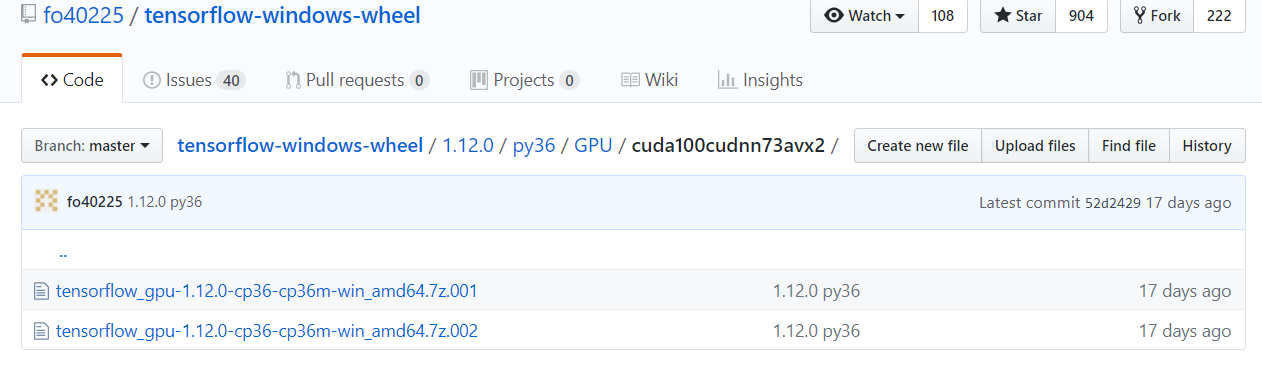VS2017+CUDA10.0+cudnn+tensorflow配置

CUDA10.0安装

1. 首先下载安装cuda官方地址，需要注册一个nvidia developer，用n卡账号登陆进去注册就行了，很简单。注册成功后会发邮件提示你注册成功。下载界面如下：安装过程全程默认即可。
2. **设置环境变量，**打开系统变量，安装过程中已经自动添加了两条环境变量，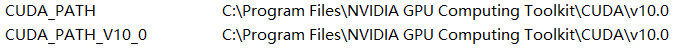之后我们再手动添加以下几条：
CUDA_SDK_PATH = C:\ProgramData\NVIDIA Corporation\CUDA Samples\v10.0
CUDA_LIB_PATH = %CUDA_PATH%\lib\x64
CUDA_BIN_PATH = %CUDA_PATH%\bin
CUDA_SDK_BIN_PATH = %CUDA_SDK_PATH%\bin\win64
CUDA_SDK_LIB_PATH = %CUDA_SDK_PATH%\common\lib\x64


1. 检测安装，安装目录下.\extras\demo_suite有deviceQuery.exe 和 bandwidthTest.exe两个exe文件，我们用cmd来运行检查安装成功与否。
在cmd界面进入cuda10的安装文件夹，键入deviceQuery.exe和bandwidthTest.exe 来运行，最后Result = PASS 即可。

VS2017配置

1. 首先新建一个cpp空项目：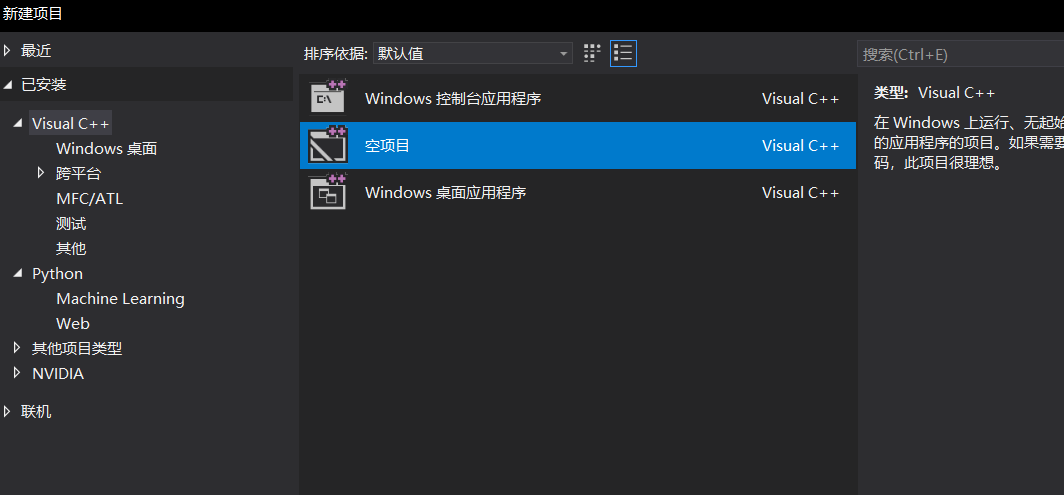2. 添加>新建项，取名cuda_main。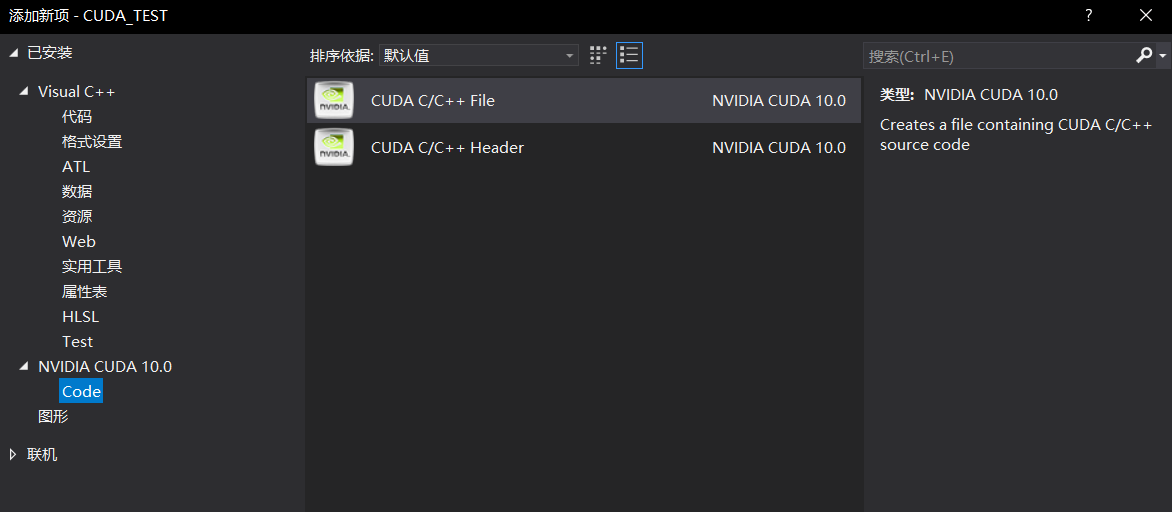3. 右键CUDA_TEST>生成依赖项>生成自定义，选择CUDA 10.0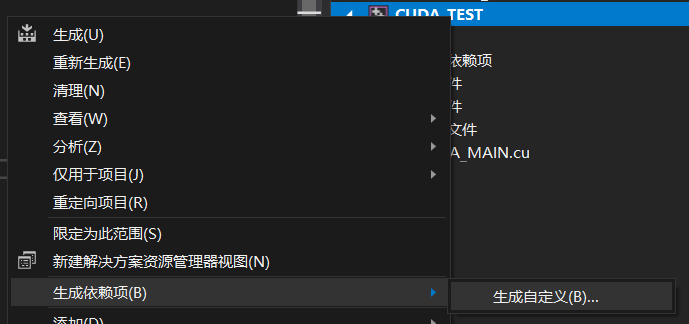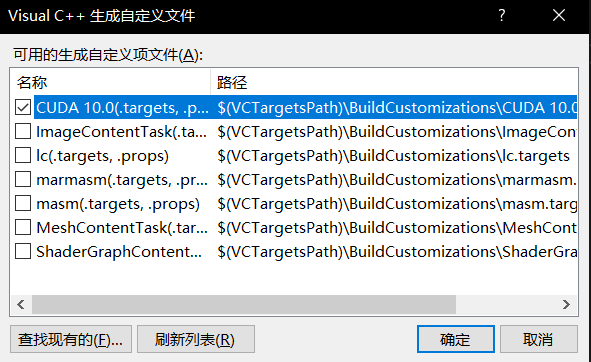4. 右键cuda_main.cu的属性,在配置属性–>常规–>项类型–>选择“CUDA C/C++”。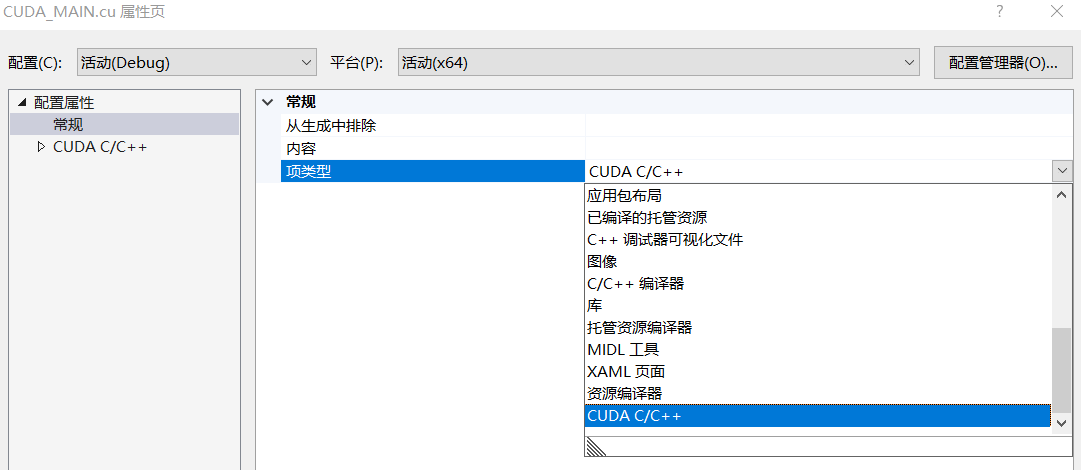项目配置：//这里照搬了笔尖bj

1.x64

1.1 包含目录配置
1.右键点击项目属性–>属性–>配置属性–>VC++目录–>包含目录
2.添加包含目录：
$(CUDA_PATH)\include 1.2 库目录配置 1.VC++目录–>库目录 2.添加库目录：$(CUDA_PATH)\lib\x64

1.3 依赖项
配置属性–>链接器–>输入–>附加依赖项
添加库文件：
cublas.lib
cuda.lib
cudart.lib
cudart_static.lib
nvcuvid.lib
OpenCL.lib

2.x86(win32)

2.1 包含目录配置

添加包含目录：
$(CUDA_PATH)\include 2.2 库目录配置 1.VC++目录–>库目录 2.添加库目录：$(CUDA_PATH)\lib\Win32

2.3 依赖项
配置属性–>连接器–>输入–>附加依赖项
添加库文件：
cuda.lib
cudart.lib
cudart_static.lib
nvcuvid.lib
OpenCL.lib

测试文件

// CUDA runtime 库 + CUBLAS 库
#include "cuda_runtime.h"
#include "cublas_v2.h"

#include <time.h>
#include <iostream>

using namespace std;

// 定义测试矩阵的维度
int const M = 5;
int const N = 10;

int main()
{
// 定义状态变量
cublasStatus_t status;

// 在 内存 中为将要计算的矩阵开辟空间
float *h_A = (float*)malloc (N*M*sizeof(float));
float *h_B = (float*)malloc (N*M*sizeof(float));

// 在 内存 中为将要存放运算结果的矩阵开辟空间
float *h_C = (float*)malloc (M*M*sizeof(float));

// 为待运算矩阵的元素赋予 0-10 范围内的随机数
for (int i=0; i<N*M; i++) {
h_A[i] = (float)(rand()%10+1);
h_B[i] = (float)(rand()%10+1);

}

// 打印待测试的矩阵
cout << "矩阵 A :" << endl;
for (int i=0; i<N*M; i++){
cout << h_A[i] << " ";
if ((i+1)%N == 0) cout << endl;
}
cout << endl;
cout << "矩阵 B :" << endl;
for (int i=0; i<N*M; i++){
cout << h_B[i] << " ";
if ((i+1)%M == 0) cout << endl;
}
cout << endl;

/*
** GPU 计算矩阵相乘
*/

// 创建并初始化 CUBLAS 库对象
cublasHandle_t handle;
status = cublasCreate(&handle);

if (status != CUBLAS_STATUS_SUCCESS)
{
if (status == CUBLAS_STATUS_NOT_INITIALIZED) {
cout << "CUBLAS 对象实例化出错" << endl;
}
getchar ();
return EXIT_FAILURE;
}

float *d_A, *d_B, *d_C;
// 在 显存 中为将要计算的矩阵开辟空间
cudaMalloc (
(void**)&d_A,    // 指向开辟的空间的指针
N*M * sizeof(float)    //　需要开辟空间的字节数
);
cudaMalloc (
(void**)&d_B,
N*M * sizeof(float)
);

// 在 显存 中为将要存放运算结果的矩阵开辟空间
cudaMalloc (
(void**)&d_C,
M*M * sizeof(float)
);

// 将矩阵数据传递进 显存 中已经开辟好了的空间
cublasSetVector (
N*M,    // 要存入显存的元素个数
sizeof(float),    // 每个元素大小
h_A,    // 主机端起始地址
1,    // 连续元素之间的存储间隔
d_A,    // GPU 端起始地址
1    // 连续元素之间的存储间隔
);
cublasSetVector (
N*M,
sizeof(float),
h_B,
1,
d_B,
1
);

// 同步函数

// 传递进矩阵相乘函数中的参数，具体含义请参考函数手册。
float a=1; float b=0;
// 矩阵相乘。该函数必然将数组解析成列优先数组
cublasSgemm (
handle,    // blas 库对象
CUBLAS_OP_T,    // 矩阵 A 属性参数
CUBLAS_OP_T,    // 矩阵 B 属性参数
M,    // A, C 的行数
M,    // B, C 的列数
N,    // A 的列数和 B 的行数
&a,    // 运算式的 α 值
d_A,    // A 在显存中的地址
N,    // lda
d_B,    // B 在显存中的地址
M,    // ldb
&b,    // 运算式的 β 值
d_C,    // C 在显存中的地址(结果矩阵)
M    // ldc
);

// 同步函数

// 从 显存 中取出运算结果至 内存中去
cublasGetVector (
M*M,    //  要取出元素的个数
sizeof(float),    // 每个元素大小
d_C,    // GPU 端起始地址
1,    // 连续元素之间的存储间隔
h_C,    // 主机端起始地址
1    // 连续元素之间的存储间隔
);

// 打印运算结果
cout << "计算结果的转置 ( (A*B)的转置 )：" << endl;

for (int i=0;i<M*M; i++){
cout << h_C[i] << " ";
if ((i+1)%M == 0) cout << endl;
}

// 清理掉使用过的内存
free (h_A);
free (h_B);
free (h_C);
cudaFree (d_A);
cudaFree (d_B);
cudaFree (d_C);

// 释放 CUBLAS 库对象
cublasDestroy (handle);

getchar();

return 0;
}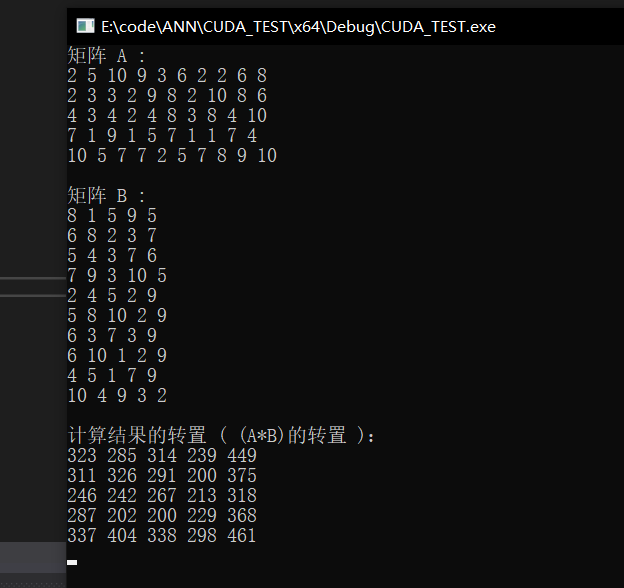cudnn和tensorflow的安装比较简单，这里一起列出

cudnn下载地址,选择 for CUDA 10.0 那个版本，之后将bin、include、lib三个文件夹覆盖粘贴至CUDA安装目录即可。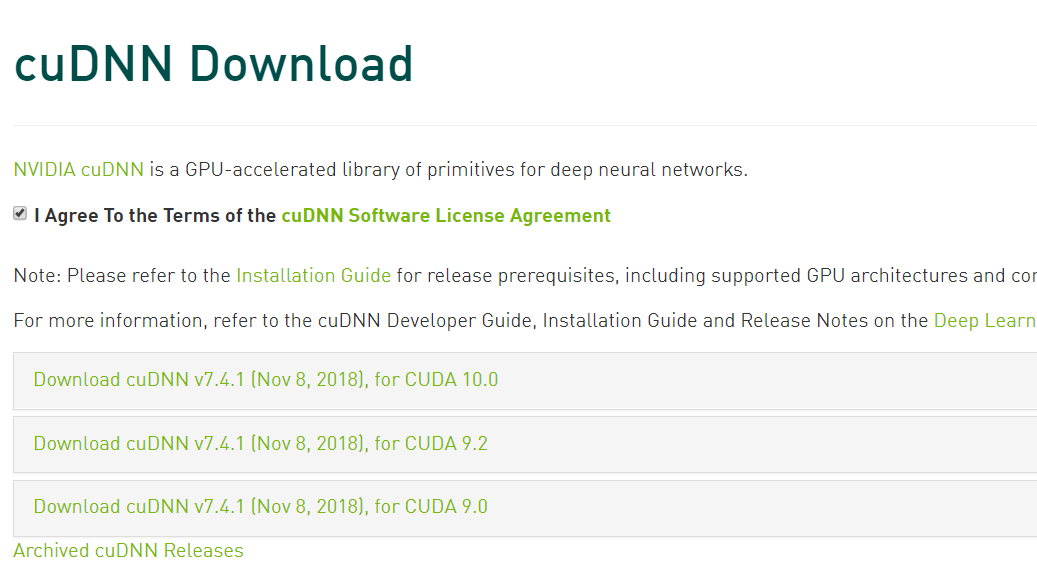tensorflow1.12-gpu 安装